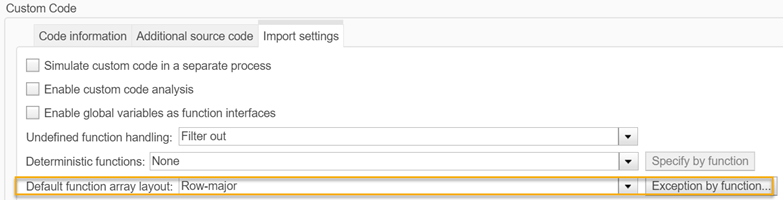# Use External Functions with Matrix Input in a C Function Block

This example shows how to pass a matrix input to a C Function block and do row-major operations using external custom code.

```open_system('mMatrixColumnSumExternalCode'); ```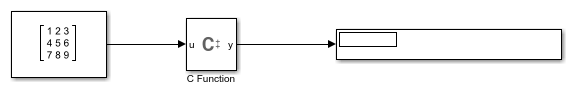In this example, a matrix input is used by external custom code to calculate the sum of the each column of the matrix and the results are passed to the output block. The external function accesses input array `arr` as row-major using `arr[r][c]`.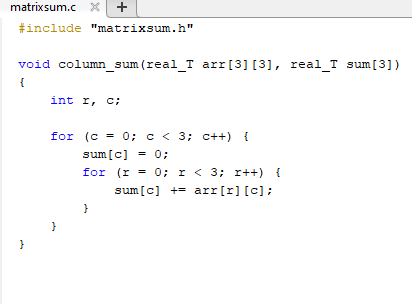The custom code is called in the Output Code pane of the C Function block.The external source and header files are specified on the Configuration Parameters > Simulation Target pane.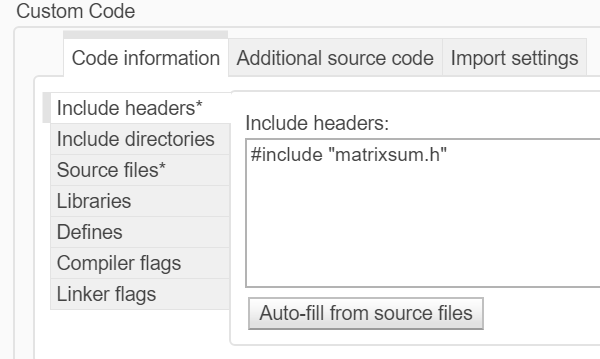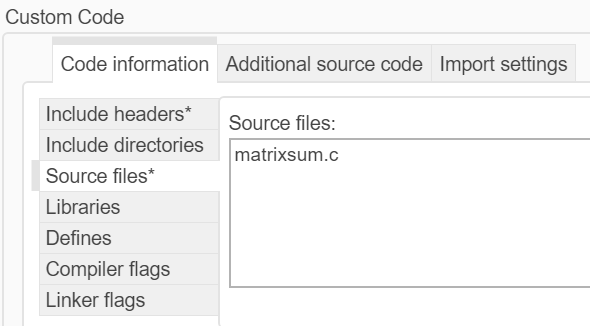For the matrix input, the size of the input symbol on the matrix table is specified as `[r c]`, where `r` corresponds to the number of rows in the matrix input and `c` corresponds to the number of columns.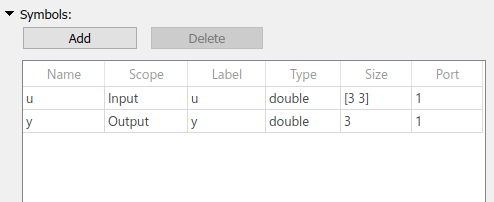### Specify the Default Function Array Layout

The external C function accesses input array `arr` using row-major layout. To ensure the validity of calculations with MATLAB, go to Default Function Array Layout under Configuration Parameters > Simulation Target and select `Row-major`.